# Research Spotlight: BMXNet – An Open Source Binary Neural Network Implementation Based On MXNet

This is guest post by Haojin Yang, Martin Fritzsche, Christian Bartz, Christoph Meinel from the Hasso-Plattner-Institut, Potsdam Germany. We are excited to see research drive practical implementation of deep learning on low power devices. This work plays an important part in expanding powerful intelligent capabilities into our everyday lives.

In recent years, deep learning technologies have achieved excellent performance and many breakthroughs both in academia and industry. However the state-of-the-art deep models are computationally expensive and consume large storage space. Deep learning is also strongly demanded by numerous applications in areas such as mobile platforms, wearable devices, autonomous robots and IoT devices. How to efficiently apply deep models on such low power devices becomes a challenging problem.

The recently proposed Binary Neural Networks (BNNs) can drastically reduce memory size and access by applying bitwise operations instead of standard arithmetic operations. By significantly improving the efficiency and lowering the energy consumption at runtime, state-of-the-art deep learning models can be implemented on low power devices. This technique combined with the developer-friendliness of OpenCL (compared to VHDL/Verilog), also makes FPGAs a viable option for deep learning.

In this post we want to introduce BMXNet, which is an open-source BNN (Binary Neural Network) library based on Apache MXNet. The developed BNN layers can be seamlessly applied with other standard library components and work in both the GPU and CPU mode. BMXNet is maintained and developed by the multimedia research group at Hasso Plattner Institute and released under Apache license. The library, several sample projects, and a collection of pre-trained binary models are available for download at https://github.com/hpi-xnor

## Framework

BMXNet provides activation, convolution and fully connected layers that support binarization of input data and weights. These layers are designed as drop-in replacements for the corresponding MXNet variants and are called QActivation, QConvolution and QFullyConnected. They provide an additional parameter act_bit, which controls the bit width calculated by the layers. A Python example usage of the proposed binary layers in comparison to MXNet is shown in listing 1 and listing 2. We do not use binary layers for the first and last layer in the network since this may greatly decrease accuracy. The standard block structure of a BNN in BMXNet is conducted as: QActivation-QConvolution or QFullyConnected-BatchNorm-Pooling as shown in the following listings.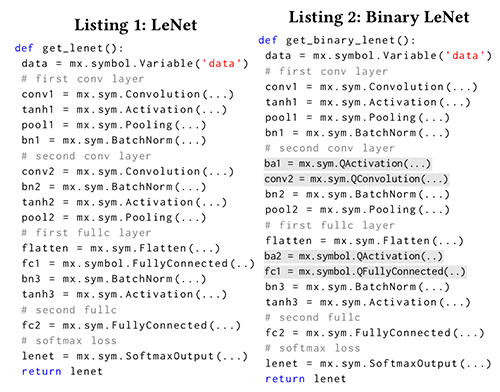In traditional Deep Learning models, fully connected and convolution layers rely heavily on dot products of matrices, which in turn require massive floating point operations. In contrast, working with binarized weights and input data allows for highly performant matrix multiplications by utilizing the CPU instructions xnor and popcount. Most modern CPUs are optimized for these types of operations. To calculate the dot product of two binary matrices A◦B, multiplication operations are no longer required. The element-wise multiplication and summation of each row of A with each column of B can be approximated by first combining them with the xnor operation and then counting the number of bits set to 1 in the result, which is the population count. This way we could take advantage of hardware support for such bitwise operations. The population count instruction is available on x86 and x64 CPUs supporting SSE4.2, while on ARM architecture it is included in the NEON instruction set. An unoptimized GEMM (General Matrix Multiplication) implementation utilizing these instructions is shown in listing 3: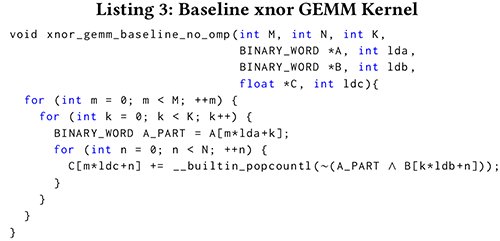The compiler intrinsic builtin popcount is supported by both gcc and clang compilers and translates into the machine instruction on supported hardware. BINARY_WORD is the packed data type storing 32 (x86 and ARMv7) or 64 (x64) matrix elements, each represented by a single bit. We implemented several optimized versions of xnor GEMM kernel, by which we tried to leverage processor cache hierarchies by blocking and packing the data, and using unrolling and parallelization techniques.

## Training and Inference

For the training stage we carefully designed the binarized layers to exactly match the output of the built-in layers of MXNet (computing with BLAS dot product operations) when limiting weights and inputs to the discrete values -1 and +1. After calculation of the dot product we map the result back to the value range of the proposed xnor style dot product, as described in following equation: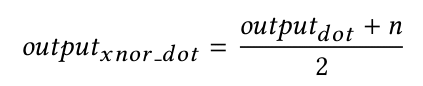where n is the value range parameter. This setting enables massively parallel training with GPU support by applying CuDNN. The trained model can then be used for doing inference on less powerful devices (without GPU support and with small storage space) where the forward pass for prediction will calculate the dot product with the xnor and popcount operations instead of standard arithmetic operations.

After training a network with BMXNet, the weights are stored in 32 bit float variables. This is also the case for networks trained with a bit width of 1 bit. We provide a model_converter that reads in a binary trained model file and packs the weights of QConvolution and QFullyConnected layers. After conversion only 1 bit of storage and runtime memory is used per weight. For instance a ResNet-18 network (on CIFAR-10) with full precision weights has a size of 44.7MB. The conversion with our model converter achieves 29× compression resulting in a file size of 1.5MB.

## Classification Accuracy

We conducted experiments with our BNNs on the MNIST (Hand written digit recognition), CIFAR-10 (image classification) datasets. The experiments were performed on a workstation that has an Intel(R) Core(TM) i7-6900K CPU, 64 GB RAM and 4 TITAN X (Pascal) GPUs.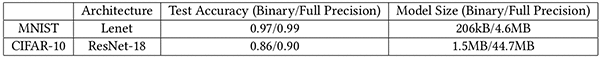The table above depicts the classification test accuracy of our binary and full precision models trained on MNIST and CIFAR-10. The table shows that the size of binary models is significantly reduced. At the same time, the accuracy is still competitive. We also conducted experiments of binarized, partially-binarized and full precision models on ImageNet dataset where the partially-binarized models show promising results while the fully binarized model still has a large improvement space (further details can be found in our paper).

## Efficiency Analysis

We conducted experiments to measure the efficiency of different GEMM methods based on Ubuntu16.04/64-bit platform with Intel 2.50GHz × 4 CPU with popcnt in-struction (SSE4.2) and 8G RAM. The measurements were performed within a convolution layer. Here, we fixed the parameters as follows: filter number=64, kernel size=5×5, batch size=200, and the matrix sizes M, N, K are 64, 12800, kernel_w × kernel_h ×inputChannelSize, respectively. The figure below shows the results.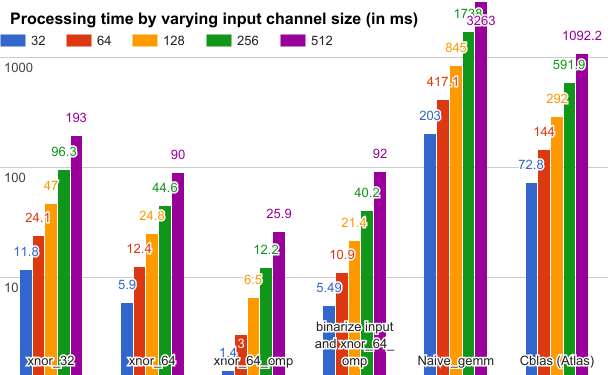The colored columns denote the processing time in milliseconds across varying input channel size: xnor_32 and xnor_64 denote the xnor gemm operator in 32 bit and 64 bit; xnor_64_omp denotes the 64 bit xnor gemm accelerated by using the OpenMP parallel programming library; binarize input and xnor_64_omp further accumulated the processing time of input data binarization. By accumulating the binarization time of input data we still achieved about 13× acceleration as compared to Cblas method.

## Conclusion

We introduced BMXNet, an open-source binary neural network implementation in C/C++ based on MXNet. In our current experiments we have achieved up to 29× model size saving and much more efficient binary gemm computation. We developed sample applications for image classification on Android as well as iOS using a binarized ResNet-18 model. Source code, documentation, pre-trained models and sample projects are published on GitHub. As the next step we would like to conduct more systematic exploration in terms of both accuracy and efficiency on different deep architectures, and re-implement the unpack_patch2col method in the Q_Conv layer to further improve the inference speed in CPU mode.